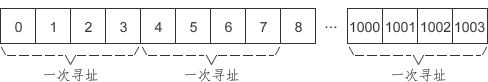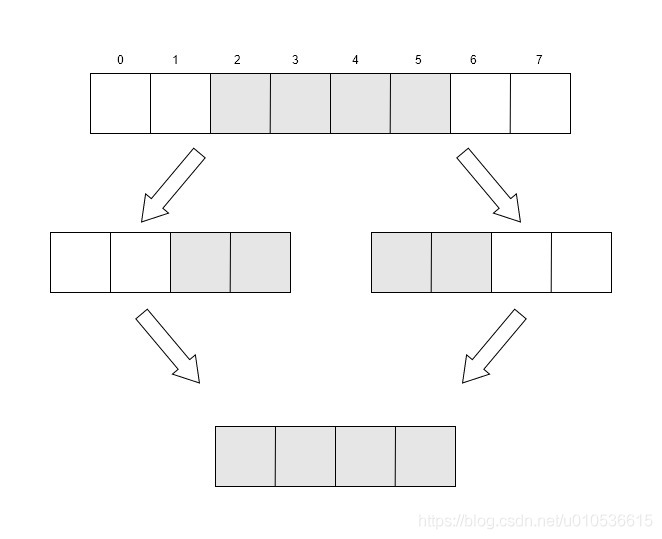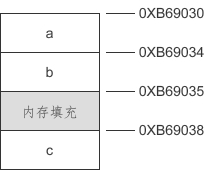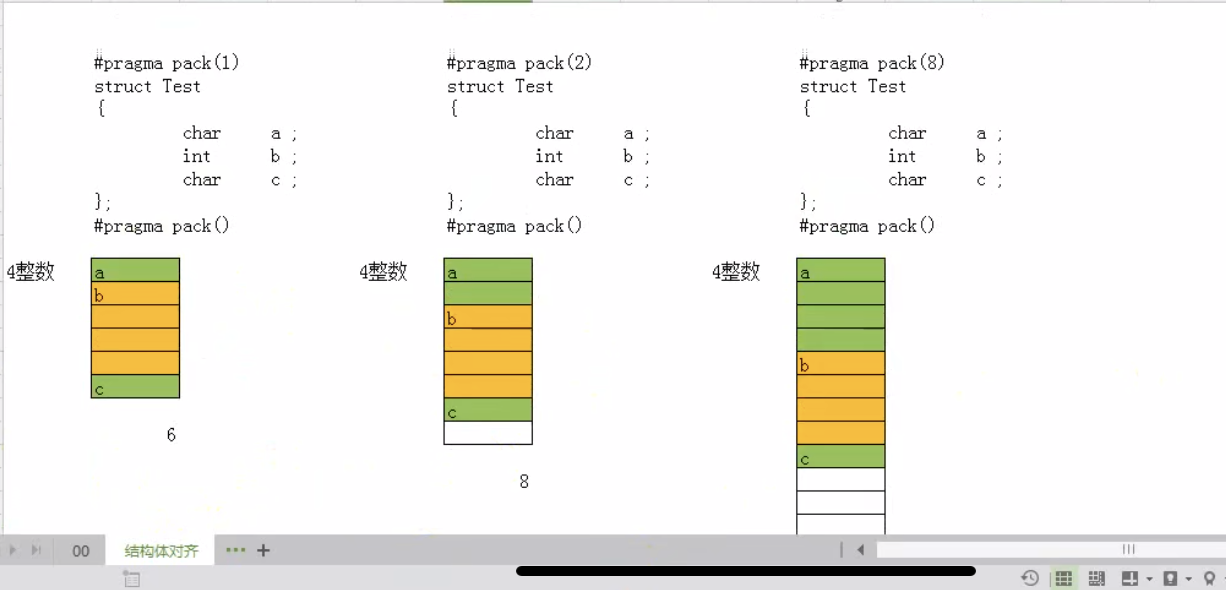Computer memory is divided in bytes, so in theory the `CPU` can access any number of bytes, but in practice this is not the case. For a CPU with a data bus width of `32 bits`, the actual addressing step is 4 bytes, which means that only memory numbered in multiples of 4 is addressed, such as `0, 4, 8, 12, 1000`, and so on, but not memory numbered `1, 3, 11, 1001`. (This is also true for 64-bit processors, which read 8 bytes at a time).

Take `32-bit CPU` addressing as an example.This allows for the fastest possible addressing: not missing a byte, and not addressing a byte repeatedly. For a program, it is best for a variable to lie within the range of one addressing step so that the value of the variable can be read at once; if it is stored across steps, it needs to be read twice and then the data is stitched together, which is obviously less efficient.

The following figure shows.When the data is in `2-5`, the `32-bit CPU` actually reads `0-3` first, then `4-7` bytes, and then merges the data obtained twice to get the required four bytes of data. Another example is a `int` type data, if the address is `8`, then it is good to address the numbered `8` memory once. Putting a piece of data within a single step to avoid storing it across steps is called memory alignment.

## Memory Alignment of Structs

To improve access efficiency, the compiler automatically performs memory alignment, see the following code.

 `````` 1 2 3 4 5 6 7 8 9 10 11 12 13 14 15 `````` ``````#include #include struct{ int a; char b; int c; }t={ 10, 'C', 20 }; int main(){ printf("length: %d\n", sizeof(t)); printf("&a: %X\n&b: %X\n&c: %X\n", &t.a, &t.b, &t.c); system("pause"); return 0; } ``````

The results of running in 32-bit compilation mode.

 ``````1 2 3 4 `````` ``````length: 12 &a: B69030 &b: B69034 &c: B69038 ``````

If memory alignment is not considered, the structure variable `t` should occupy `4+1+4 = 9` bytes of memory. Considering memory alignment, although member `b` only occupies `1` byte, there are still `3` bytes left in the addressing step where it is located, and there is no room for an `int` type variable, so we have to put member `c` in the next addressing step. The remaining `3` bytes are wasted as memory fill.The reason why the compiler wants memory alignment is to access member c more efficiently, at the cost of wasting 3 bytes of space.

The above example shows that the starting address of a structure variable needs to be divisible by the width of its own variable, and if it cannot, it needs to be filled with bytes in front, and there is another rule when calculating the size of a structure, the total size of the structure must be divisible by the size of the widest member, and if it cannot, it is filled with bytes in the back.

## Global Variable Memory Alignment

In addition to structs, variables are also memory aligned, see the following code.

 `````` 1 2 3 4 5 6 7 8 9 10 `````` ``````#include #include int m; char c; int n; int main(){ printf("&m: %X\n&c: %X\n&n: %X\n", &m, &c, &n); system("pause"); return 0; } ``````

Running results.

 ``````1 2 3 `````` ``````&m: DE3384 &c: DE338C &n: DE3388 ``````

Byte alignment: If a variable takes up `n` bytes, the starting address of the variable must be an integer multiple of `n`, i.e.: `Starting storage address % n = 0`, with bytes added if not enough.

You can see that they are all addressed as integer multiples of 4 and next to each other. Although memory alignment is hardware related, it is the compiler that determines the alignment. If your hardware is 64-bit but compiled in 32-bit, it will still be aligned according to 4 bytes.

The alignment can be modified by compiler parameters.

## Set alignment factor

In case of high memory requirements, we can drop space-for-time in favor of time-for-space, where we customize the alignment instead of following all the compiler default alignment, and change the alignment of the structure members by `#pragma pack(n)`. `n` can be defined as `1, 2, 4, 8, 16`.

Let’s see the example.

 `````` 1 2 3 4 5 6 7 8 9 10 11 12 13 14 15 16 `````` ``````// 我们通过切换系数查看不同占用大小 #pragma pack(1) struct Struct1 { char a;//1byte int b;//4byte char c;//1byte } t; #pragma pack() int main(){ printf("length: %d\n", sizeof(t)); printf("&a: %X\n&b: %X\n&c: %X\n", &t.a, &t.b, &t.c); return 0; } ``````

Output:

 ``````1 2 3 4 `````` ``````length: 6 &a: 407970 &b: 407971 &c: 407975 ``````As you can see from the figure above, the memory footprint size does change after the coefficients are changed. And the rule is mainly: the variable width is compared with the alignment factor, and whoever is smaller uses which for alignment. For example, when `pack(1)`, `a` occupies `1 byte`, `b` needs `4 bytes`, but the comparison with the coefficient is greater than the coefficient, so the alignment is done according to `1 byte`, which is next to the memory of `a`, and `c` is the same.

Factor N = Min(maximum member width, alignment factor), of course the overall size of the structure must also be an integer multiple of N, not an integer multiple need to make up bytes.